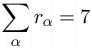## Day 1, Problem 1: Scribble

Nixed, he placed the flong into the calathi halfway through the yuga.

Huh?

Believe it or not, the above sentence is actually a valid English sentence. It also has two other features: it looks like spam, and the words are very valuable.

Valuable, you say? (For some reason, you are doing lots of talking to yourself today).

Yes, valuable, if you are playing Scribble. In the standard game of Scribble, calathi (which means "a vase-shaped basket represented in Greek painting and sculpture") is worth 72 points, nixed (meaning "refused") is worth 26 points, flong (which is "a compressed mass of paper sheets, forming a matrix or mold for stereotype plates") is worth 18 points, and yuga which is "any one of the four ages, Krita, or Satya, Treta, Dwapara, and Kali, into which the Hindus divide the duration or existence of the world" is worth 33 points.

Specifically, as you may know, each letter in Scribble is worth a given number of points. The goal is get the most points with a given set of letters.

For this question, we will modify the game slightly. Suppose you have 7 tiles/letters and you have scores for each letter (where the score sα for each letter α satistisfies 0 < sα < 26), and also you have a dictionary of valid words that you can consult before you play (this is different than the "normal" Scribble play). Your task is the find the highest scoring word.

### Input

You are given a number k (1 ≤ k ≤ 7) on the first line of input. On the next k lines, you will be given triples α sα rα, where α is a letter, sα is the score for that letter, and rα is the number of times that letter occurs as a tile. You can assume that$\displaystyle \sum_{\alpha}r_{\alpha} = 7$

For example, the triple "a 7 2" means you have two tiles marked a and each is worth 7 points. On the next (the k + 2nd) line, there is the number N (0 < N < 100000). On each of the next N lines, there is a word (you can assume the length of each word is at least one).

### Output

The output one line long, containing one integer, which is the maximum score. That is, the maximum number of points that can be attained by using the tiles to form one complete word. If no word can be formed, the maximum number of points is zero.

### Sample Input

4
a 1 1
b 4 1
c 2 1
d 10 4
3
ab
bc
c

### Sample Output

6

Point Value: 10
Time Limit: 2.00s
Memory Limit: 16M

Languages Allowed:
C++03, PAS, C, HASK, ASM, RUBY, PYTH2, JAVA, PHP, SCM, CAML, PERL, C#, C++11, PYTH3

• (1/0)
In the 9th test case, N is 10, but there are only 9 following words...
Also, there is a similar situation in the 3rd test case.
It may not affect C++ submissions, but it had certainly screwed my Python program...

• (0/0)
Roger that. I'll check with Troy, but this was so long ago that the original test file probably doesn't exist anywhere anymore. So I'll probably just remove this test file entirely.

• (0/0)
I confirm that test cases 3 and 9 have something weird about them.

In submission 180781, I terminate early if there are no more lines to read, resulting in no output for cases 3 and 9.

In submission 180782, I immediately print out my best result if there is no input to read, which gives AC.

• (0/0)
Test cases 3 and 9 are missing some words. btw how do you check if there are no more lines to read?

Edit: nvm I got it

• (0/0)
I fixed those cases and rejudged.

• (0/0)
Thanks! :)

• (2/0)
Ignore the sigma. I did.
Basically, you're given a list of letters, their point value, and the number of them in the game. i.e.
a 1 1 b 4 1 c 2 1 d 10 4
means that there is one occurrence of letter a, each worth one point; there is one occurrence of letter b, each worth four points; there is one occurrence of letter c, each worth two points; and there are four occurrences of letter d, each worth ten points.

You're then given a list of words. Your job is to find the highest scoring word, given the letter scores. i.e.,
ab // worth 5 points (a + b = 1 + 4 = 5) bc // worth 6 points (b + c = 4 + 2 = 6) c  // worth 2 points (c = 2)

You would output 6, as this is the maximum score attainable.

• (1/0)
It says that the sum of all the "r"s will be equal to 7.
(Or, there will always be exactly 7 tiles in your hand)

• (1/0)
Naturally, yes.

• (0/1)
It's what totally screwed me up....

• (0/0)
not really
I was just asking to possibly make my program better/faster
of course, yours is accepted and mine isn't so...

• (1/2)

• (0/0)
I'm pretty sure it doesn't matter...

• (2/0)
Just use a string - it won't be that slow.
And yes the letters are all lowercase.

• (0/1)